Processing ......FreeComputerBooks.com Links to Free Computer, Mathematics, Technical Books all over the World

Higher Algebra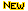Top Free Data Science Books - 100% Free or Open Source!
• Title Higher Algebra
• Author(s) Jacob Lurie
• Publisher: Harvard University (2017)
• Hardcover/Paperback N/A
• eBook PDF (1553 pages)
• Language: English
• ISBN-10: N/A
• ISBN-13: N/A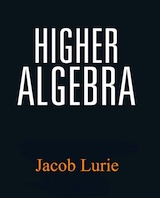Book Description

on

This book is an informal and readable introduction to higher algebra at the post-calculus level with focus on Stable infinite-Categories; infinite-Operads; Algebras and Modules over infinte-Operads; Associative Algebras and Their Modules; Little Cubes and Factorizable Sheaves; Algebraic Structures on infinite-Categories; Algebra in the Stable Homotopy Category; Constructible Sheaves and Exit Paths; Categorical Patterns.

• Jacob Lurie is a professor at Department of Mathematics, Harvard University, Cambridge.
Reviews, Ratings, and Recommendations: Related Book Categories: Read and Download Links:Similar Books:
•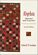Algebra: Abstract and Concrete (Frederick M. Goodman)

This introduction to modern or abstract algebra addresses the conventional topics of groups, rings, and fields with symmetry as a unifying theme, while it introduces readers to the active practice of mathematics.

•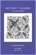Abstract Algebra: Theory and Applications (Thomas W Judson)

This book is designed to teach the principles and theory of abstract algebra to college juniors and seniors in a rigorous manner. Its strengths include a wide range of exercises, both computational and theoretical, plus many nontrivial applications.

•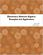Elementary Abstract Algebra: Examples and Applications

It introduces the basic notions of group theory by a thorough treatment of important examples, including complex numbers, modular arithmetic, symmetries, and permutations, also applications to communications, cryptography, and coding theory.

•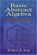Basic Abstract Algebra (Robert B. Ash)

This book surveys fundamental algebraic structures and maps between these structures. Its techniques are used in many areas of mathematics, with applications to physics, engineering, and computer science as well.

•Set Theoretic Approach to Algebraic Structures in Mathematics

This book brings out how sets in algebraic structures can be used to construct the most generalized algebraic structures, like set linear algebra / vector space, set ideals in groups and rings and semigroups, and topological set vector spaces.

•Advanced Algebra (Anthony W. Knapp)

This book is on modern algebra which treat various topics in commutative and noncommutative algebra and provide introductions to the theory of associative algebras, homological algebras, algebraic number theory, and algebraic geometry.

•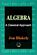Algebra: A Classical Approach (Jon Blakely)

This book provides a complete and contemporary perspective on classical polynomial algebra through the exploration of how it was developed and how it exists today, with discrete explanations from functions to quadratic and linear equations, etc.

•Linear Algebra (Jim Hefferon)

This textbook covers linear systems and Gauss' method, vector spaces, linear maps and matrices, determinants, and eigenvectors and eigenvalues. Each chapter has three or four discussions of additional topics and applications.

•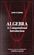Algebra: A Computational Introduction (John Scherk)

This book is a unique approach and presentation, the author demonstrates how software can be used as a problem-solving tool for algebra. It includes many computations, both as examples and as exercises.

•A Computational Introduction to Number Theory and Algebra

This introductory book emphasises algorithms and applications, such as cryptography and error correcting codes, and is accessible to a broad audience. It covers the basics of number theory, abstract algebra and discrete probability theory.

•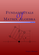Fundamentals of Matrix Algebra (Gregory Hartman)

A college (or advanced high school) level text dealing with the basic principles of matrix and linear algebra. It covers solving systems of linear equations, matrix arithmetic, the determinant, eigenvalues, and linear transformations.

•Computational Linear Algebra and N-dimensional Geometry

This undergraduate textbook on Linear Algebra and n-Dimensional Geometry, in a self-teaching style, is invaluable for sophomore level undergraduates in mathematics, engineering, business, and the sciences.

Book Categories
 :All CategoriesTop BooksRecent BooksMiscellaneous BooksComputer LanguagesComputer ScienceData Science/DatabasesElectrical EngineeringJava and Java EE (J2EE)Linux and UnixMathematicsMicrosoft and .NETMobile ComputingNetworking and CommunicationsSoftware EngineeringSpecial TopicsWeb Programming
Other Categories